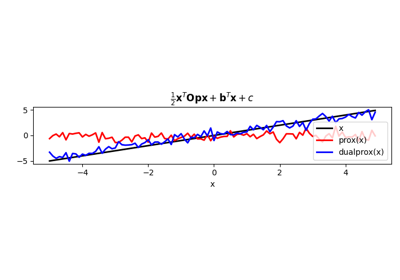class pyproximal.Quadratic(Op=None, b=None, c=0.0, niter=10, x0=None, warm=True)[source]#

Proximal operator for a quadratic function: $$f(\mathbf{x}) = \frac{1}{2} \mathbf{x}^T \mathbf{Op} \mathbf{x} + \mathbf{b}^T \mathbf{x} + c$$.

Parameters
Oppylops.LinearOperator, optional

Linear operator (must be square)

bnp.ndarray, optional

Vector

cfloat, optional

Scalar

niterint, optional

Number of iterations of iterative scheme used to compute the proximal

x0np.ndarray, optional

Initial vector

warmbool, optional

Warm start (True) or not (False). Uses estimate from previous call of prox method.

Raises
ValueError

If Op is not square

Notes

The Quadratic proximal operator is defined as:

$\prox_{\tau f}(\mathbf{x}) = \left(\mathbf{I} + \tau \mathbf{Op} \right)^{-1} \left(\mathbf{x} - \tau \mathbf{b}\right)$

when both Op and b are provided. This formula shows that the proximal operator requires the solution of an inverse problem. If the operator Op is of kind explicit=True, we can solve this problem directly. On the other hand if Op is of kind explicit=False, an iterative solver is employed. In this case it is possible to provide a warm start via the x0 input parameter.

When only b is provided, the proximal operator reduces to:

$\prox_{\mathbf{b}^T \mathbf{x} + c}(\mathbf{x}) = \mathbf{x} - \tau \mathbf{b}$

Finally if also b is not provided, the proximal operator of a constant function simply becomes $$\prox_c(\mathbf{x}) = \mathbf{x}$$

Methods

 __init__([Op, b, c, niter, x0, warm]) affine_addition(v) Affine addition chain(g) Chain grad(x) Compute gradient postcomposition(sigma) Postcomposition precomposition(a, b) Precomposition prox(**kwargs) proxdual(**kwargs)

## Examples using pyproximal.Quadratic#